H alg II mod 6 Quiz Polynomials Practice name multiple Choice

 Date 16.06.2018 Size 410.47 Kb. #52198
H Alg II Mod 6 Quiz Polynomials Practice NAME _____________________
Multiple Choice

Identify the choice that best completes the statement or answers the question.

____ 1. Which of the following is a factor of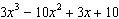?
 A B C D x +2

____ 2. Which of the following is NOT a factor of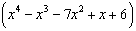?

 A C B D

____ 3. What is the remainder when is divided by ?

 A C B D 25

____ 4. For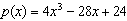, . Which of the following must therefore be true?

 A is a factor of. B is a factor of. C is a factor of. D is a factor of.

____ 5. Divide: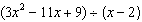A C B D

____ 6. In the expression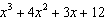, when the first two terms are grouped, and the last two terms are grouped, what is the common binomial factor?

 A C B D

____ 7. What is the complete factorization of ?

 A C B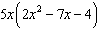D

____ 8. Factor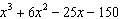.

 A C () B () D

____ 9. Divide.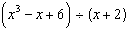A C B D

____ 10. As a first step in factoring , substitute x for . What is the resulting trinomial expression?

 A C B D

Multiple Response

Identify one or more choices that best complete the statement or answer the question.
____ 1. Use the remainder theorem and the factor theorem to determine which of the following binomials are factors of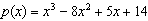.

 A B C D E F

1. Use the remainder theorem and the factor theorem to show that is a factor of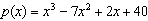. Then factorcompletely.

2. Divide.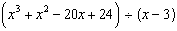3. Rewrite the expression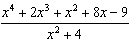as the sum of a polynomial and a rational expression whose numerator is a constant. Show your work. (Long Division)

4. Factor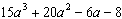by grouping.

5. Divide.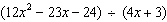Essay
1. Is a factor of ? How do you know?

Other
1. Use the remainder theorem and the factor theorem to determine whether the given binomial is a factor of the given polynomial.

 a. ; Yes No b. ; Yes No

H Alg II Mod 6 Quiz Polynomials Practice

MULTIPLE CHOICE
1. ANS: A PTS: 1 DIF: DOK 1 NAT: A-APR.B.2
2. ANS: B PTS: 1 DIF: DOK 2 NAT: A-APR.B.2
3. ANS: B PTS: 1 DIF: DOK 1 NAT: A-APR.B.2
4. ANS: D

The factor theorem says that for a polynomial and a number a, if and only if is a factor of . Since for this , must be a factor of .

 Feedback__A'>Feedback A You cannot factor from the terms of . B You cannot factor from the terms of . C The factor theorem tells you that when , is a factor of . D That’s correct!

PTS: 1 DIF: DOK 1 NAT: A-APR.B.2 KEY: remainder theorem | factor theorem

5. ANS: B PTS: 1 DIF: DOK 1

OBJ: Using Synthetic Division to Divide by a Linear Binomial NAT: A-APR.D.6

LOC: MTH.C.10.05.08.03.03.003 TOP: Dividing Polynomials
6. ANS: C PTS: 1 DIF: DOK 1 NAT: A-SSE.A.1b

KEY: complicated expressions

7. ANS: C PTS: 1 DIF: DOK 1 NAT: A-SSE.A.2
8. ANS: D PTS: 1 DIF: DOK 2 OBJ: Factoring by Grouping

NAT: A-SSE.A.2 LOC: MTH.C.10.05.08.03.04.011 TOP: Factoring Polynomials

9. ANS: B PTS: 1 DIF: DOK 1 NAT: A-APR.D.6
10. ANS: B PTS: 1 DIF: DOK 1 NAT: A-SSE.A.1b

KEY: complicated expressions

MULTIPLE RESPONSE
1. ANS: A, D, F

The remainder theorem says that for a polynomial and a number a, the remainder on division of by is . The factor theorem states that if and only if is a factor of .

A: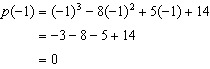B: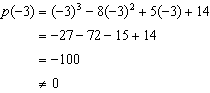C: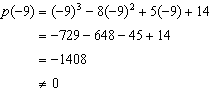D: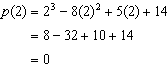E: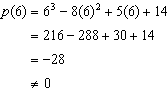F: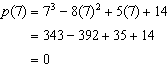Feedback Correct That’s correct! Incorrect To see whether is a factor of , find and then use the remainder theorem and the factor theorem.

PTS: 2 DIF: DOK 1 NAT: A-APR.B.2 KEY: remainder theorem | factor theorem

1. ANS:

Use synthetic substitution to find :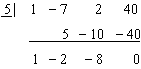The last number in the bottom row of the synthetic substitution array is . Since , is a factor of . The coefficients of the quotient when is divided by are the first three numbers in the bottom row of the synthetic substitution array. So,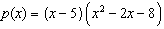. Factoring the quadratic factor by inspection gives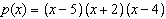, the complete factorization of .
Rubric

1 point for showing is a factor of;

1 point for finding as a factor;

1 point for finding as a factor

PTS: 3 DIF: DOK 2 NAT: A-APR.B.2

KEY: remainder theorem | factor theorem | synthetic substitution | factoring

2. ANS:

PTS: 1 DIF: DOK 1 NAT: A-APR.D.6

3. ANS: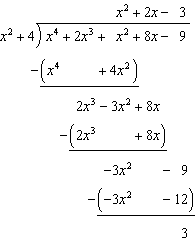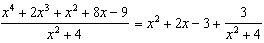Rubric

1 point for dividing correctly;

1 point for rewriting the expression in the correct form

PTS: 2 DIF: DOK 1 NAT: A-APR.D.6

KEY: rational expressions | dividing polynomials
4. ANS: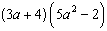PTS: 1 DIF: DOK 1 NAT: A-SSE.A.2

5. ANS:

PTS: 1 DIF: DOK 1 NAT: A-APR.D.6

LOC: NCTM.PSSM.00.MTH.9-12.ALG.2.c KEY: polynomial division
ESSAY
1. ANS:

If a polynomial simplifies to 0 when a is substituted for x, then is a factor of the polynomial.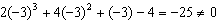is not a factor of .

PTS: 1 DIF: DOK 2 NAT: A-APR.B.2
OTHER
1. ANS:

a. No

b. Yes

c. Yes

d. No

e. Yes

PTS: 2 DIF: DOK 1 NAT: A-APR.B.2 KEY: remainder theorem | factor theorem